Time: 3 Hours                                                                                                     Max. Marks: 100

NOTE: There are 9 Questions in all.

·      Question 1 is compulsory and carries 20 marks. Answer to Q. 1. must be written in the space provided for it in the answer book supplied and nowhere else.

·      Out of the remaining EIGHT Questions answer any FIVE Questions. Each question carries 16 marks.

·      Any required data not explicitly given, may be suitably assumed and stated.

Q.1       Choose the correct or best alternative in the following:                                         (2x10)

a.       The second degree Taylor series polynomial expansion of the function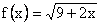about x = 0 is

(A)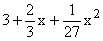.                       (B)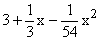.

(C)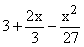.                            (D)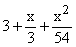.

b.      A root of the equation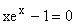lies in the interval (0.5, 1.0).  The number of iterations required by the bisection method to obtain this root correct to 2 decimal places is

(A)    13.                                               (B)  11.

(C) 9.                                                  (D)  7.

c.       Newton-Raphson method is used to obtain the approximate value of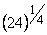.  If the initial approximation is taken as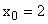, this the value obtained after two iterations is

(A)    2.5.                                              (B)  2.25.

(C)  2.2142.                                        (D)  2.1242.

d.   The homogeneous system of equationshas

(A)    two parameter family of solutions.

(B)    one parameter family of solutions.

(C)  only zero solution.

(D)  no solution.

e.   The spectral radius of the Gauss-Jacobi iteration method to solve the linear system of equationsis

(A)     1.26.                                            (B)  1.50.

(C)  2.00.                                            (D)  2.50.

f.    The data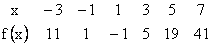represents an nth degree polynomial.  The value of n is

(A)     4.                                                 (B)  3.

(C)  2.                                                 (D)  1.

g.   The truncation error in the method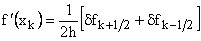is

(A)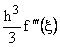.                                    (B)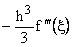.

(C)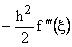.                                 (D)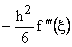.

h.   The value of the integral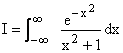using two point Gauss-Hermite method is

(A)    0.5908.                                        (B) 1.1813.

(C)  2.0944.                                        (D) 4.1888.

i.    The value of b in the formula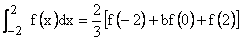is

(A)   1.                                                 (B) 2.

(C) 4.                                                  (D) 8.

j.    The approximate value of y(1.4) for the initial value problem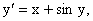y(1) = 2

obtained by using the Euler’s method with h=0.2 is

(A)  2.5472.                                        (B)  2.7596.

(C)  2.7637.                                        (D) 2.9253.

Answer any FIVE Questions out of EIGHT Questions.

Each question carries 16 marks.

Q.2     a.   Derive the secant method to determine a simple root of the equation f(x) = 0.  Find its rate of convergence.                                                            (8)

b.   Find an interval of unit length which contains the smallest negative root in magnitude of the equation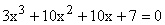(i)                  Perform two iterations of the bisection method.

(ii)                Taking end points of the last interval as initial approximations, perform two iterations of the secant method.            (8)

Q.3       a.   Perform two iterations of the Newton’s method to solve the system of equations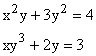Take the initial approximation as (0.8, 0.9).                                                      (10)

b.   Find all solutions of the system of equationsusing Gauss-elimination method.                                                                       (6)

Q.4      a.     Set up the Gauss-Seidel iteration method in matrix form to solve the linear system of equationsFind its rate of convergence, if the method is convergent.                      (8)

b.    Find the inverse of the matrixusing Choleski method.                                                                                (8)

Q.5     a.       Using five iterations of the inverse power method, find the eigenvalue which is nearest to 4 in magnitude for the matrix.Take the initial approximate eigenvector as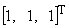.                            (8)

b.      Using Given’s method, reduce the matrixto tri-diagonal form.  Obtain the Sturm’s sequence and hence all the eigenvalues.         (8)

Q.6    a.   Prove the following operator relations

(i)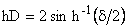(ii)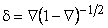(4)

b.      Using Newtonts divided difference interpolation, obtain the polynomial

which fits the data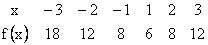(6)

c.   Obtain the least squares approximation of the form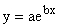for the data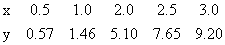(6)

Q.7   a.  Obtain the maximum absolute truncation error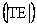and the maximum

absolute round off error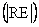in the method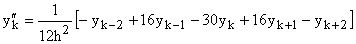where

(i)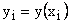for all i

(ii)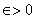is the maximum round off error in evaluating y(x) for all x

(iii)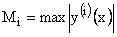for all x in the given interval.

Determine the optimal value of h so that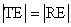.                                                (8)

b.    Obtain the Richardson’s extrapolation scheme for the method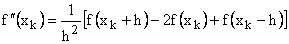Using this method and Richardson’s extrapolation, find the best value of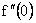using the data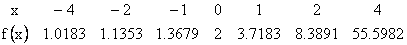(8)

Q.8   a.  Find the minimum number of intervals required to evaluate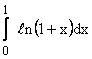using the Simpson’s rule with an accuracy of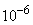.                         (8)

b.    Obtain the values of the constants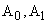and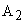in the method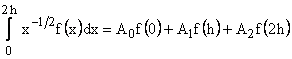where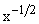is the weight function, so that the method is of highest possible

order.  Find the order and the error term of the method.                                             (8)

Q.9   a.      Solve the initial value problem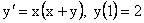in the interval [1, 1.4] using the classical fourth order Runge-Kutta method with h=0.2.                                       (8)

b.   Using the multi-step method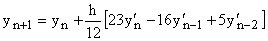with h = 0.2, obtain the approximate value of y(1.8) for the initial value problem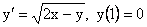Find the starting values using the Euler method with h = 0.2                                         (8)